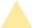• Language
Request A Quote
Featured Blog

## GEO Data Mining (II) – Differential gene expression analysis and visualization with complete code sharing

Why is differential expression analysis of genes valuable? During tumor pathogenesis or the progress of other diseases, gene expression can change. Some genes that were originally silent may become highly expressed, while other genes that were originally expressed normally may have increased or decreased expression in experimental samples. Genes that have altered expression levels compared with normal samples may control or affect tumorigenesis. Therefore, to study tumorigenesis mechanisms, investigators need to set up case (experiment or treatment) and control (control) groups for DGE analysis, explore the differentially expressed genes that characterize the treatment group and the control group, and construct gene enrichment pathways to study the pathogenesis of tumors. Then investigators can focus on interpreting these enriched pathways and explore the relationship or internal mechanism between these pathways and the phenotype of the sample.

Through the following four steps, we present a complete set of shared code for differential gene expression analysis and visualization: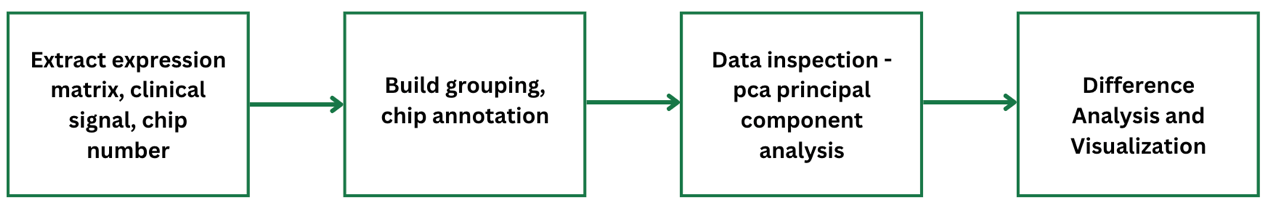Step 1: Extract expression matrix, clinical information, and chip number

The original data we downloaded, including metadata, constitutes the “eSet”. We will use the method of subsetting the list to extract the first element in the eSet list: eSet[]. We will also use the “exprs” function to convert it into a matrix, usually using head (100) to determine whether the data needs to be converted to log type.

#(1) Extract expression matrix exp—-

exp <- exprs(eSet[])
exp[1:4,1:4]
#exp = log2(exp+1) #Judge whether log2 is needed

#(2) Extract clinical information —-
The clinical information of each sample can be obtained by using the pData() function. Usually, the source column (source) or feature column (characteristics”) of the data frame will describe which samples are control or treatment.

pd <- pData(eSet[])

#(3) Extract chip platform number —-
The data usually use different chip probes, which are subsequently converted into entrez ID or symbol ID according to the GEO Platform (GPL) number. The NCBI database’s official name for the gene is represented by the Entrez ID. The HGNC database’s official name for the gene is represented by the symbol ID.

gpl <- eSet[]@annotation
p = identical(rownames(pd),colnames(exp))
save(gse,exp,pd,gpl,file = “step1_output.Rdata”)#Save the result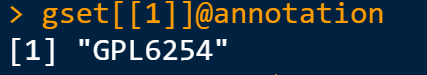Step 2: Build grouping, chip annotation

1. Build group information
The Rdata from Step 1 are grouped according to the sample source information in the GEO Series (GSE).

rm(list = ls())
library(stringr)
table(colnames(exp))
group_list = ifelse(str_detect(pd\$source_name_ch1,”tumor”),”test”,”normal”)
group_list=factor(group_list,
levels = c(“test”,”normal”))
group_list
table(group_list)

2. Chip annotation of GLP

if(T){
a = getGEO(gpl,destdir = “.”)
b = a@dataTable@table
colnames(b)
ids2 = b[,c(“ID”,”GENE_SYMBOL”)]
colnames(ids2) = c(“probe_id”,”symbol”)
ids2 = ids2[ids2\$symbol!=”” & !str_detect(ids2\$symbol,”///”),]
}
save(group_list,ids2,exp,pd,file = “step2_output.Rdata”)

Step 3: Data inspection – Principal Component Analysis

Next, perform principal component analysis (PCA) with the FactoMineR, factoextra package to determine whether there is a significant grouping between treatment and control groups. Use the code below to draw a PCA plot.

#Import the Rdata results of the first two steps

rm(list = ls())#clear the environment
dat=as.data.frame(t(exp))
library(FactoMineR)
library(factoextra)
# Unified operation of pca
dat.pca <- PCA(dat, graph = FALSE)
pca_plot <- fviz_pca_ind(dat.pca,
geom.ind = “point”, # show points only (nbut not “text”)
col.ind = group_list, # color by groups
palette = c(“#00AFBB”, “#E7B800”),
addEllipses = TRUE, # Concentration ellipses
legend.title = “Groups”
)
print(pca_plot)
ggsave(plot = pca_plot, filename = paste0(gse,”PCA.png”))
save(pca_plot, file = “pca_plot. Rdata”)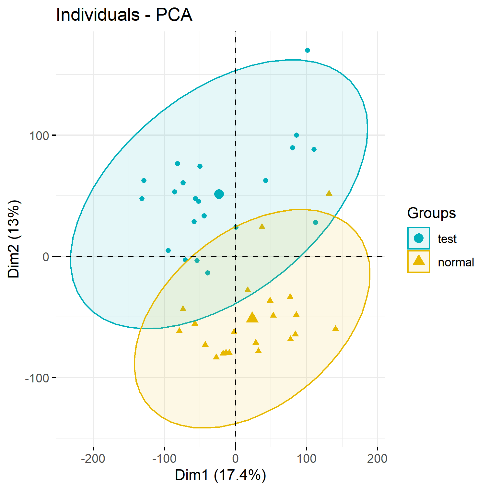PCA plots（significant difference between subgroups of data）

Step 4: Differential gene expression analysis and visualization – volcano map and heat map

Next, import the Rdata from Steps 1 and 2, and use the limma package to perform differential gene expression (DGE) analysis on each gene. DGE analysis includes determining log fold change (logFC) of a gene, average expression level, and whether the p value is significant. DGE analysis uses two main input files: (1) the organized expression matrix, in which the row name is the gene name and the column name is the sample name; and (2) grouping information (group list).

DGE analysis with the limma package is divided into three steps: lmFit, eBayes, and topTable. Using the limma package requires three types of data: (1) Expression matrix exp grouping matrix; (2) Design difference comparison matrix; and (3) Contrast.matrix to get the differential analysis matrix (nrDEG). The analysis results focus on logFC and p value.

rm(list = ls())

1. Differential gene expression analysis

library(limma)
design=model.matrix(~group_list)
fit=lmFit(exp, design)
fit=eBayes(fit)
deg=topTable(fit, coef=2, number = Inf)

2. Add a few columns to the deg data frame

#1. Add the probe_id column and turn the row name into a column
library(dplyr)
deg <- mutate(deg,probe_id=rownames(deg))
#tibble::rownames_to_column()
#merge merges two tables
table(deg\$probe_id %in% ids\$probe_id)
#deg ​​<- inner_join(deg,ids,by="probe_id")
deg <- merge(x = deg,y = ids2, by="probe_id")
deg <- deg[!duplicated(deg\$symbol),]
dim(deg)
#2. Add change column: up or down, the volcano map should be used
#logFC_t=mean(deg\$logFC)+2*sd(deg\$logFC)
logFC_t=1.5
change=ifelse(deg\$P.Value>0.05,’stable’,
ifelse( deg\$logFC >logFC_t,’up’,
ifelse( deg\$logFC < -logFC_t,'down','stable') )
)
deg <- mutate(deg, change)
table(deg\$change)

3. Add the ENTREZID column, which will be used later in the enrichment analysis

library(ggplot2)
library(clusterProfiler)
library(org.Hs.eg.db)
s2e <- bitr(unique(deg\$symbol), fromType = "SYMBOL",
toType = c( “ENTREZID”),
OrgDb = org.Hs.eg.db)
deg <- inner_join(deg,s2e,by=c("symbol"="SYMBOL"))
save(logFC_t,deg,file = “step4_output.Rdata”)

Data visualization- volcano map, heat map

Several different types of graphs are available to visualize differential gene expression data, including volcano maps and heat maps.

rm(list = ls())
library(dplyr)

1.Volcano map

dat <- mutate(deg,v=-log10(P.Value))
if(T){
for_label <- dat%>%
filter(symbol %in% c(“RUNX2″,”FN1”))
}
if(F){
}
if(F) {
x1 = dat %>%
filter(change == “up”) %>%
x2 = dat %>%
filter(change == “down”) %>%
for_label = rbind(x1,x2)
}
p <- ggplot(data = dat,
aes(x = logFC,
y = v)) +
geom_point(alpha=0.4, size=3.5,
aes(color=change)) +
ylab(“-log10(Pvalue)”)+
scale_color_manual(values=c(“blue”, “grey”,”red”))+
geom_vline(xintercept=c(-logFC_t,logFC_t),lty=4,col=”black”,lwd=0.8) +
geom_hline(yintercept = -log10(0.05), lty=4, col=”black”, lwd=0.8) +
theme_bw()
p
volcano_plot <- p +
geom_point(size = 3, shape = 1, data = for_label) +
ggrepel::geom_label_repel(
aes(label = symbol),
data = for_label,
color=”black”
)
volcano_plot
ggsave(plot = volcano_plot, filename = paste0(gse,”volcano.png”))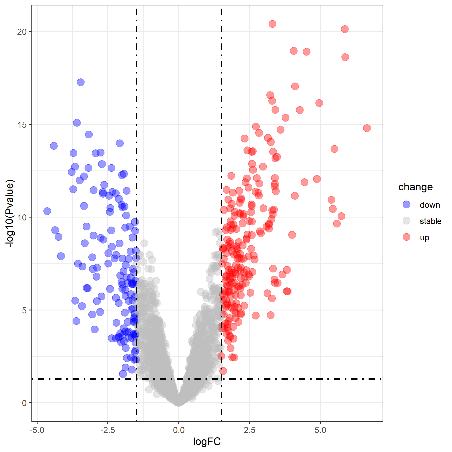Volcano plot（Upregulation & Downregulation in Gene Expression）

2. Draw a heatmap

cg=names(tail(sort(apply(exp,1,sd)),1000))
n=exp[cg,]
annotation_col=data.frame(group=group_list)
rownames(annotation_col) = colnames(n)
library(pheatmap)
heatmap_plot <- pheatmap(n,
show_colnames=F,
show_rownames = F,
annotation_col = annotation_col,
scale = “row”)
# save the result
library(ggplot2)
png(file = paste0(gse,”heatmap.png”))
ggsave(plot = heatmap_plot, filename = paste0(gse,”heatmap.png”))
dev.off()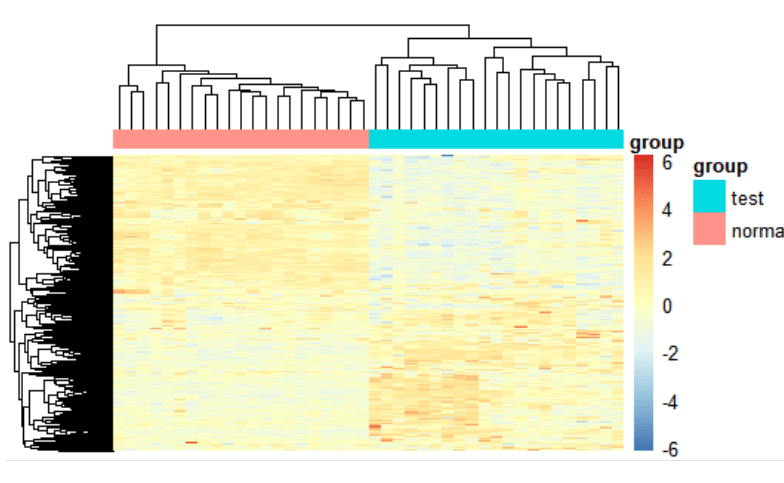Heatmap（Measuring similarity of expression between subgroups）

At present, we have completed the differential gene expression analysis and visualization part of GEO data mining and have obtained significantly up-regulated and down-regulated differentially expressed genes. However, disease occurrence is often affected by more than one gene. For more tools to assist in studying disease and cancer mechanisms, stay tuned for our next tutorial on GEO data mining and identifying gene expression pathways.

## More Resources